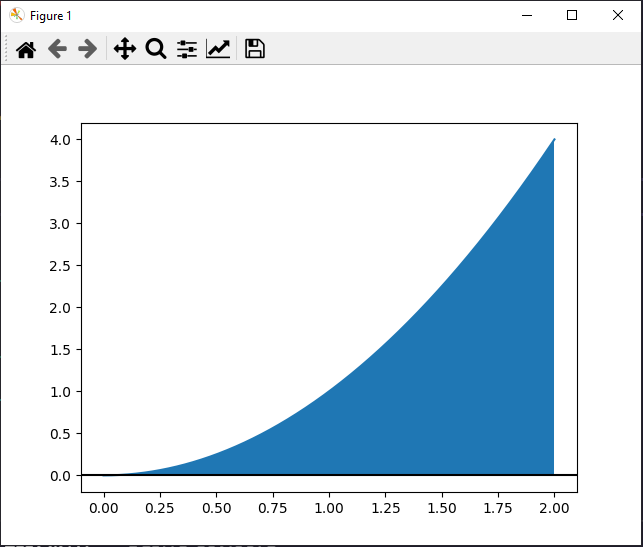Skip to content
Related Articles
How to find Definite Integral using Python ?
• Difficulty Level : Basic
• Last Updated : 15 Mar, 2021

Definite integrals are the extension after indefinite integrals, definite integrals have limits [a, b]. It gives the area of a curve bounded between given limits.It denotes the area of curve F(x) bounded between a and b, where a is the lower limit and b is the upper limit.

In this article, we will discuss how we can solve definite integrals in python, and would also visualize the area between them using matplotlib. We would also use the NumPy module for defining the range of the variable we are integrating. Let’s Begin with installing the modules.

### Module needed:

• matplotlib: We would use this to visualize our area under the graph formed by a definite integral.
• numpy: Helper library to define ranges of definite integrals.
• sympy: Library to calculate the numerical solution of the integral easily.

### Approach

For calculating area under curve

• Import module
• Declare function
• Integrate.

Syntax :

sympy.integrate(expression, reference variable)

For plotting

• Import module
• Define a function
• Define a variable
• Draw the curve
• Fill the color under it using some condition.
• Display plot

Given below is the implementation for the same.

The area between a curve and standard axis

Example 1 :

## Python

 import matplotlib.pyplot as pltimport numpy as npimport sympy as sy    def f(x):    return x**2  x = sy.Symbol("x")print(sy.integrate(f(x), (x, 0, 2)))

Output:

8/3

Example 2:

## Python3

 import matplotlib.pyplot as pltimport numpy as np    def f(x):    return x**2  x = np.linspace(0, 2, 1000)plt.plot(x, f(x))plt.axhline(color="black")plt.fill_between(x, f(x), where=[(x > 0) and (x < 2) for x in x])plt.show()

Output:The area between two curves

Example 1:

## Python3

 import matplotlib.pyplot as pltimport numpy as npimport sympy as sy    def f(x):    return x**2    def g(x):    return x**(1/2)    x = sy.Symbol("x")print(sy.integrate(f(x)-g(x), (x, 0, 2)))

Output:

0.781048583502540

Example 2:

## Python3

 import matplotlib.pyplot as pltimport numpy as np    def f(x):    return x**2    def g(x):    return x**(1/2)    x = np.linspace(0, 2, 1000)plt.plot(x, f(x))plt.plot(x, g(x))plt.fill_between(x, f(x), g(x), where=[(x > 0) and (x < 2) for x in x])plt.show()

Output:Attention geek! Strengthen your foundations with the Python Programming Foundation Course and learn the basics.

To begin with, your interview preparations Enhance your Data Structures concepts with the Python DS Course. And to begin with your Machine Learning Journey, join the Machine Learning – Basic Level Course

My Personal Notes arrow_drop_up